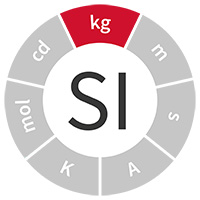Close
Measurement for Recovery
Close
Measurement for Recovery

SI units

# kilogram (kg)

The kilogram is the SI unit of massAccurately measuring the mass of an object is essential in many applications, from administering the optimum dose of a drug to correctly manufacturing materials with the desired properties.

For more than a hundred years we compared the gravitational force on an object with the gravitational force on a reference piece of metal known as a 'standard weight'. The standard weight was in turn compared with the International Prototype of the Kilogram (IPK), held in the International Bureau of Weights and Measures in France, which was the ultimate reference for all mass measurements. However, the value of the IPK may have changed since it was produced in 1884; contamination, cleaning or just time may have increased or decreased its mass.

Since the revision of the SI on 20 May 2019, we can now compare the gravitational force on an object with an electromagnetic force using a Kibble balance. This allows the kilogram to be defined in term of a fixed numerical value of the Planck constant, a constant which will not change over time.

## Definition

The kilogram is defined by taking the fixed numerical value of the Planck constant, ℎ, to be 6.626 070 15 × 10-34 when expressed in the unit J s, which is equal to kg m2 s−1, where the metre and the second are defined in terms of the speed of light, 𝒸, and the hyperfine transition frequency of the caesium-133 atom, ∆ν, respectively.

This was a new definition in May 2019.

Application

NPL provides traceability for the measurement of active components of drugs that can weigh less than a millionth of a kilogram to oil sea platforms that can weigh over 200 million kilograms.

## Did you know?

• At 4 °C a 10 cm cube of water has a mass of a kilogram, and a 1 m cube of water has a mass of approximately one tonne
• NPL is home to copy number 18 of the International Prototype of the Kilogram
• The Kibble balance was developed at NPL in the UK by Dr Bryan Kibble after whom it is named

The science behind the unit

The kilogram was the last base unit to be defined by a physical object. Science and industry required a more accurate and stable way to measure mass, so the new definition of the kilogram is in terms of a fundamental constant of nature, which improves its long-term stability. It also eliminates the necessity for traceability to a single physical artefact, so the mass scale can theoretically be realised at any location and at any value, not just at 1 kilogram.

NPL has developed the Kibble balance, which balances gravitational force with an electromagnetic force.

The weight of a mass is balanced against the force generated by a current-carrying coil held in a magnetic field. The ratio of the force generated by the coil to the current passing through it is calibrated in a second phase of the experiment, which measures the voltage generated by the coil as it is moved at a measured velocity through the magnetic field. As the voltage and the current are measured using quantum electrical standards, the kilogram can be defined in terms of a fixed value of the Planck constant, h, plus the existing definitions of the metre and the second. The ultimate target is to measure a kilogram with an accuracy of a millionth of one percent, every time.

Find out about NPL's research in this area